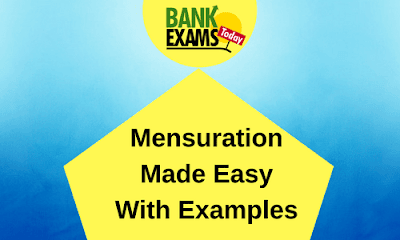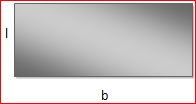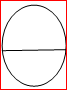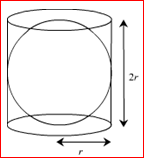# Mensuration Made Easy With Examples## Introduction:

We usually make faces when asked to go through the topic ”MENSURATION”, the sole reason behind this is “THE FORMULAS” but wait what if we had to just understand everything and apply it in practical life ?? Read it right, today we are going to get our basic concepts cleared under this topic with few examples supporting the concepts.

Before heading to the formulas, let's know the meaning of the word MENSURATION. The word mensuration means measurement, this is a branch of mathematics which helps us in dealing with the study of plane and solid figures, their area, volume, and related parameters.

## Important Definitions

### 1. Area

The extent or measurement of a surface or piece of land or the total amount of space inside the boundary of flat objects is Area. The shaded region in the following image is the area of the rectangle.### 2. Perimeter

The perimeter of any 2-dimensional object is the measure of the covering the inner area.
Here the dotted lines represent the boundary
If
→ l =10cm and b=4 then perimeter = 2 (l+b)
→ 2(10+4)
→ 2 x14
→ 28 cm (add the boundary measurements you get 28)### 3. Circumference

When you have any curved geometric figure then their distance around the body will be called as circumference, especially for the circle.

### 4. Dimensional Objects

A three-dimensional shape is a solid shape that has height and depth. For example, a sphere and a cube are three-dimensional, but a circle and a square are not.

### 5. Curved Surface Area:

The surfaces which are not flat, are called curved surface.
The lateral surface is the area of the vertical faces of the solid.
Curved surfaces do not include the top or bottom are, for example, CSA of the cylinder will not include the area of upper and lower circle.
Whereas the total surface area (TSA) will include both the area of both upper and bottom portion.

• Area of a square = side x side = (side)2
• Perimeter of square will be total of its four sides measurement = 4(side)
• Area of a rectangle = l x b (similar to square where we multiple sides of the same measurement but rectangle has two different measurements )
• The perimeter of a rectangle = 2 (l+b) or simply add the sides.
• Perimeter of a circle = 2⊓r
• Area of a circle = ⊓r2 (where r is the radius )

### Now, what is ⊓?

⊓ is the relationship between distance around the circle i.e. (circumference) and distance across the circle whose value is 22/7 or 3.14……..
For any circle, the ratio remains same no matter how big or small it is (radius will not matter)
Example:
D stands for diameter (2 x radius = diameter)

D=7
Circumference=22D=14
circumference= 44D=21
circumference=66So comparing the relationship between circumference of all these circles we get same value
→ C1/D1= 22/7
→ C2/D2=44/14=22/7
Circumference may increase but the ratio remains same.
In simple terms, if you wrap the diameter of any circle to its outer boundary you will need approximately 3.14….. times diameter.

## Formulae For Different Shapes

### CylindersVolume of Cylinder = ⊓r2h
Suppose if someone asks you to find the area of a coin you can simply use the formula of circle neglecting the height of the coin. Same way if someone asks you to keep one coin over the another and ask you to calculate the area then you will have to consider the number of coins or say the height of the cylinder you will form out of the coins.
The base circle area is ⊓r2 multiplied with h will give you total volume.
Curved Surface Area of a cylinder= perimeter of base x  height = 2⊓rh
(take the circumference of the bottom circle multiply it h, will give you the total curved surface area)
Total Surface Area = Curved Surface Area + 2 base x height
Total surface area will be total of curved surface area and the base and the upper circle area which are of the same area so take either of the two base or upper)

## CONEIt takes the volume of three cones to equal one cylinder, all having the same height and radii of a cylinder.
So when volume of cylinder = ⊓r2h
Then the volume of cone = 1/3 x ⊓r2h
Curved Surface Area of cone: ⊓rl
→ H = height
→ L=slanting height which you can calculate using
→ l2 = r2 + h2

In cone, the circle circumference is 2⊓r and r is the radius
when a cone is opened, you get a shape of an arc making an imaginary circle with L as radius because L is the slant height and edge of the cone and for the imaginary circle the circumference is 2⊓l and area of the circle will be ⊓l²
Comparing their ratios will give you the area:Circumference                                    Area
Circle                   2⊓l                                                  ⊓l2
Their Ratio = ⊓l2 / 2⊓l
Circumference of arc = 2⊓r
Area of arc = ⊓l2 / 2⊓πl  x 2⊓r = ⊓rl
Curved Surface Area = ⊓rl
Total Surface Area  = Curved Surface Area + area of base circle
= ⊓ r l + ⊓r= ⊓ r ( r+l )

## SPHERE:A sphere is a perfectly round geometrical object in
three- dimensional space, best example cricket ball or football. spheres do not have any bottom or top is totally curvy. So both lateral/curved surface and total surface are equal

Total Surface Area = Curved Surface Area
It becomes difficult to find area of curvy objects so with help of cylinder we can understand this: (proved by greek mathematician Archimedes)
You can fit a sphere in cylinder (with same radii and height)
Then the lateral surface of cylinder = area of sphere
Curved Surface Area of Cylinder = 2⊓rh
Curved Surface Area of sphere = 2⊓rh
( r is same for both, we do not have h in sphere so we consider diameter to be h =2r)
Curved Surface Area = 2⊓r(2r) substituting h=2r
Curved Surface Area= 4⊓r2
Volume of sphere =4/3⊓r3 (sphere is 1/3rd of cursed surface of sphere into r)
Volume of hemisphere = 2/3 2⊓r3 (half of the volume of sphere )
Curved Surface Area of hemisphere = 4⊓r2/ 2 (half of spheres) = 2⊓r2NOTERemember friends TSA of a hemisphere is not same as CSA (don’t do this mistake of halving the TSA of the sphere because hemisphere has one side circle so you will have to add the area of the circle too:
→Total Surface Area = 2⊓r2 + ⊓r= 3⊓r2

## CUBOIDIt is 3 dimensional, has length, breadth and height.
Volume of cuboid = l x b x h
Total Surface Area = 2( l x b+ b x h + h x l)

## CUBEIt is a form of the cuboid but all its sides all equal, best example can be a dice.same formula for cuboid and cube but in the cube all sides are same so, substitute we get
Volume = (l x b x h) suppose a is the side = (a x a x a) =a³
Total Surface Area = 2(a x a +a x a +a x a) = 2(3a2) = 6a²

Exercise with Solutions

### Question1

A road roller of diameter 1.75m and length 1m has to press a ground of area 1100 SQ. meter .how many revolutions does it make?

#### Solution:

We know a roller is in the shape of a cylinder, now when you roll a cylinder one revolution it makes will be equal to the curved surface area of the cylinder (you cannot take volume here because it not about how much the cylinder holds in it nor it is about the total surface of cylinder)
r=1.75/2 h=1
Area covered in one revolution= curved surface area = 2⊓rh
Total area to be pressed =1100 SQ meter
Number of revolutions= Total area to be pressed/curved surface area
= 1100 / 2⊓rh (substituting value )
Ans: -Number of revolutions = 200

### Question2

A rectangular sheet of paper of length 10 cm and breadth 24cm is rolled end to end to form a right circular cylinder of height 8 cm. find the volume of the cylinder.

#### Solution:

When you roll a rectangle sheet to a cylinder shape, the base forms a circle. We need to find out what the circumference of this circle is to get the radius, which will later be utilised in volume.
Circumference = 2⊓r (here clearly they have given 10 must be the height so 24 is the circumference)
→24 = 2*22/7*r
→24*7/22*1/2=r
→r = 42/11cm
Ans:-volume = ⊓r2h = 458.18 cu.cm

### Question3

A right cylindrical vessel of 15 cm radius is filled with water. Solid spheres of diameter 6 cm are dropped one by one into it. The spheres are dropped until the water level in the vessel rises by 8 cm. then, how many solid spheres are dropped into the vessel?

#### Solution:

Let n balls be dropped into the cylinder
Volume of n balls will be the total increase in water level in the vessel
Given d=6 ;so r = 3 ; rise in water level h=8
→N*4/3*⊓r3 = ⊓r2h
→N*4/3 ⊓ (3)3 = ⊓ * 152 *8
Ans:-→N= 50 balls

### Question4

If a cone and a sphere have equal radii and have equal volumes, then what is the ratio between the height of the cone and diameter of the sphere?

#### Solution:

Let h be the height of the cone and r be the radius of the sphere as well as the radius of the base of the cone, clearly given that volume of the sphere = volume of the cone
→i.e. 4/3 ⊓r3 = 1/3 ⊓r2 h
→2r=h/2=d
Therefore the height of the cone diameter of the sphere
4r:2r
Ans:-required ratio 4:2= 2:1

### Question5

The length of a rectangular plot is 60% more than its breadth. if the difference between the length and the breadth of that rectangle is 24cm. what is the area of that rectangle?

#### Solution:

Let breadth = x cm then length= 160*x/100 = 8/5*x
→So 8/5*x – x = 24
→X=40
Ans:-Then area will be =64*40= 2560

### Question6

A spherical ball of 6cm diameter is melted into a cone with base 12cm in diameter. find its height.

#### Solution:

Here diameter is given so radius will be d/2
→4/3⊓r3 = 1/3⊓R2h
→4*33 = 62 * h
Ans:-→H= 3cm

### Question7

4 containers are in the shape of a sphere of radius 7cm. find the cost of panting at Rs.2 per square metre and filling them with a liquid costing Rs.9per cubic cm.

#### Solution:

Surface area of the sphere = 4⊓r2
Surface area of the containers =4*22/7*72
→= 616 cm2
Cost of painting =Rs.2 * 616(surface area )= Rs.1232
For filling the containers you need to calculate the volume of the sphere using the formula 4/3⊓r3
Ans:-Cost of filling = Rs.9* volume of sphere = Rs.9*4/3*⊓*73 = Rs.12936#### What's trending in BankExamsToday

Smart Prep Kit for Banking Exams by Ramandeep Singh - Download here